Test: Engineering Mathematics- 5

# Test: Engineering Mathematics- 5

Test Description

## 10 Questions MCQ Test GATE Computer Science Engineering(CSE) 2023 Mock Test Series | Test: Engineering Mathematics- 5

Test: Engineering Mathematics- 5 for Computer Science Engineering (CSE) 2023 is part of GATE Computer Science Engineering(CSE) 2023 Mock Test Series preparation. The Test: Engineering Mathematics- 5 questions and answers have been prepared according to the Computer Science Engineering (CSE) exam syllabus.The Test: Engineering Mathematics- 5 MCQs are made for Computer Science Engineering (CSE) 2023 Exam. Find important definitions, questions, notes, meanings, examples, exercises, MCQs and online tests for Test: Engineering Mathematics- 5 below.
Solutions of Test: Engineering Mathematics- 5 questions in English are available as part of our GATE Computer Science Engineering(CSE) 2023 Mock Test Series for Computer Science Engineering (CSE) & Test: Engineering Mathematics- 5 solutions in Hindi for GATE Computer Science Engineering(CSE) 2023 Mock Test Series course. Download more important topics, notes, lectures and mock test series for Computer Science Engineering (CSE) Exam by signing up for free. Attempt Test: Engineering Mathematics- 5 | 10 questions in 30 minutes | Mock test for Computer Science Engineering (CSE) preparation | Free important questions MCQ to study GATE Computer Science Engineering(CSE) 2023 Mock Test Series for Computer Science Engineering (CSE) Exam | Download free PDF with solutions
 1 Crore+ students have signed up on EduRev. Have you?
Test: Engineering Mathematics- 5 - Question 1

### As xx varies from −1 to +3, which one of the following describes the behaviour of the function f(x) = x3–3x2 + 1?

Detailed Solution for Test: Engineering Mathematics- 5 - Question 1

Given x various from –1 to +3
f(x) = x3 – 3x2 + 1

f′(x) = 3x2 – 6x

⇒ f′(−1) = 3(−1) 2 – 6 (−1) = 3 + 6 = 9 > 0,f′(1) = 3 – 6 < 0

f′(0) = 0,f′(2) = 3(4) – 6(2) = 0,

f′(3) = 3x2 – 6x = 3(a) – 6(3) = 0

∴ f(x) increases, then decreases and increases again

Test: Engineering Mathematics- 5 - Question 2

### Given the following statements about a function f : R→R, select the right option: P: If f(x) is continuous at x = x0, then it is also differentiable at x = x0 Q: If f(x) is continuous at x = x0, then it may not be differentiable at x = x0 R: If f(x) is differentiable at x = x0, then it is also continuous at x = x0

Detailed Solution for Test: Engineering Mathematics- 5 - Question 2

If the function is differentiable then always it is continuous but vice – versa is not true. If the function is continuous then it may (or) may not differentiable.

*Answer can only contain numeric values
Test: Engineering Mathematics- 5 - Question 3

### Given f(x) = x3 + ax2 + bx + c and y-intercept for the function is 1. Also f has local extrema at x = -4 and x = 2. Then a + b + c = ____

Detailed Solution for Test: Engineering Mathematics- 5 - Question 3

(i) As y-intercept is 1, c = 1

(ii) Also at x = −4,f′(x) = 0

3(−4)2+2a(−4)+b+1=0

48 – 8a + b + 1 = 0

49 – 8a + b = 0

(iii) Similarly 3(2)2 + 2a(2) + b + 1 = 0

12 + 4a + b + 1 = 0

13 + 4a + b = 0

∴ a = 3, b = -24

Test: Engineering Mathematics- 5 - Question 4

Find C of Rolle’s theorem for f(x) = ex(sin x - cos x) in

Detailed Solution for Test: Engineering Mathematics- 5 - Question 4

Given function f(x) = ex(sin x - cos x)

f(x) is continuous in 3π/4

f(x) is differentiable in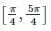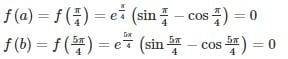f(a) = f(b)

According to Rolle’s theorem,

there exists at least one value C ∈ (a,b)

such that f’(c) = 0

f’(c) = 0

f’(x) = ex (sin x – cos x) + ex (cos x + sin x)

f’(c) = ec (sin c – cos c) + ec (cos c + sin c) = 0

⇒ 2 ec sin c = 0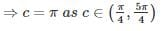Test: Engineering Mathematics- 5 - Question 5

If f(x) is differentiable and g’(x) ≠ 0 such that f(1) = 4, f(2) = 16, f’(x)= 8g’(x) and g(2) = 4 then what is the value of g(1) ?

Detailed Solution for Test: Engineering Mathematics- 5 - Question 5

By using Cauchy’s Mean value theorem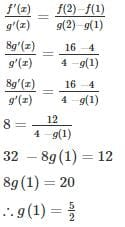Test: Engineering Mathematics- 5 - Question 6

An open box is to be made out of square cardboard of 18 cm by cutting offs equal squares from the corners and turning up the sides. What is the maximum volume of the box in cm3?

Detailed Solution for Test: Engineering Mathematics- 5 - Question 6

Let x be the corner side which is cut off and v be volume.
v = l × b × h

v = (18 − 2x) × (18 − 2x) × x

v = 4x3 − 72x2 + 324x

v′ = 12x− 144x + 324v′

v′′ = 24x − 144

v′ = 12x2 − 144x + 324 = 0

x2 − 12x + 27=0

(x−3) (x−9) = 0

(x=3) or (x = 9)

atx = 3

v′= 24x −144 = 24(3) −144 = −72<0

at x = 3 we have volume maximum

v = 4(3)3 − 72(2)2 + 324(2)

∴ v = 432

Test: Engineering Mathematics- 5 - Question 7

What are the minimum and maximum value of the below-given function respectively?

f(x) = 3x3 − 9x2 − 27x + 30

Detailed Solution for Test: Engineering Mathematics- 5 - Question 7

f(x) = 3x3 − 9x2 − 27x + 30

f′(x) = 9x2 − 18x − 27

f′′(x) = 18x − 18
Points at which maximum or minimum exists
f′(x) = 0

9x2 − 18x − 27 = 0

x2 − 2x − 3 = 0

(x − 3) (x + 1 ) = 0

(x = 3) or (x = −1)

Substitute the value in (x = 3) or (x = −1)

f′′(−1) = 18(−1) − 18 = − 36 < 0

∴ at x = −1,maximum value exists

f(−1) = 3(−1)−9(−1)−27(−1) + 30

f (−1) = 45

f′′ (3) = 18(3) − 18 = 36 > 0

∴ at x = 3, mimimum value exists

f(3) = 3(3)− 9(3)− 27(3) + 30

f (3) = −51

Test: Engineering Mathematics- 5 - Question 8

Find the point at which maximum value of f(x) = 2x3−3x2 occurs in interval [-2, 2]?

Detailed Solution for Test: Engineering Mathematics- 5 - Question 8

f(x) = 2x3 − 3x2

f′(x) = 6x2 − 6x

f′′(x) = 12x − 6

f′(x) = 6x2 − 6x = 0

6x (x−1) = 0

x = 0 or x = 1
Now check f(x) at x = 0, x = 1 and the end points of given interval since at end point tangent cannot be drawn so, above concept cannot be used to find maximum or minimum value for end points.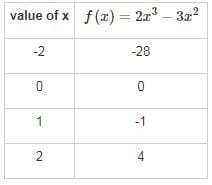Test: Engineering Mathematics- 5 - Question 9

If f(x) = x3 − 3x−1 is continuous in the closed interval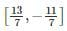and f’(x) exists in the open intervalthen find the value of c such that it lies inDetailed Solution for Test: Engineering Mathematics- 5 - Question 9

Since given function is continuous and differentiable then by Lagrange’s Mean-Value Theorem.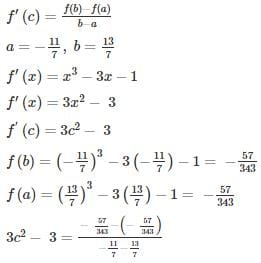3c2 − 3 = 0

c2 = 1

∴ c = ±1

Test: Engineering Mathematics- 5 - Question 10Find the maximum and minimum values of f(x) = sin x + cos 2x where 0≤ x ≤2π

Detailed Solution for Test: Engineering Mathematics- 5 - Question 10

f(x) = sin x + cos 2x in [0, 2π]

f’(x) = cos x – 2 sin 2x = cos x [1 – 4 sinx] = 0

⇒ cos x = 0; 1 – 4sinx = 0

⇒ x = π/2, 3π/2 in [0, 2π]

Sin x = 1/4, Also f’(x) exists for all x in [0, 2π]

Now f(0) = 1; f(2π) = 1; f(π/2) = 0; f(3π/2) = -2

At sinx = 1/4 , f(x) = 1/2 + (1 – 2(1/4)2) = 9/8

Therefore the maximum and minimum values of f(x) are 9/8 and -2 occurred at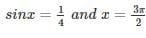## GATE Computer Science Engineering(CSE) 2023 Mock Test Series

150 docs|215 tests
Information about Test: Engineering Mathematics- 5 Page
In this test you can find the Exam questions for Test: Engineering Mathematics- 5 solved & explained in the simplest way possible. Besides giving Questions and answers for Test: Engineering Mathematics- 5, EduRev gives you an ample number of Online tests for practice

## GATE Computer Science Engineering(CSE) 2023 Mock Test Series

150 docs|215 tests(Scan QR code)